## 1. Introduction

In this tutorial, we’re going to learn about an efficient sorting algorithm called Quicksort.

Quicksort is a popular in-place sorting algorithm that makes effective use of the “Divide and Conquer” concept. It’s commonly used for sorting problems and is widely adopted as the sorting method of choice in many language libraries.

## 2. How to Sort Using Quicksort

Quicksort relies on repeating the following steps on a set of elements until they are sorted:
• Pick an element as a pivot
• Partition the problem set by moving smaller elements to the left of the pivot and larger elements to its right
• Repeat the above steps on each partition

### 2.1. Quicksort in Action

Here we have an array of ten unsorted values which we’re going to sort using Quicksort: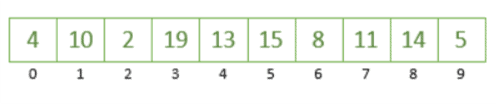The first step we’re going to take is to choose an element from this array as our pivot. We can actually pick a pivot in different ways but for this example, we’ll always pick the rightmost element of the array which is the number 5.

Now that we have determined 5 as our pivot, let’s partition the array based on our pivot by putting the numbers greater than 5 to the right and the numbers smaller than 5 to the left. At this point, we’re not really worried about ordering the numbers, just that we’ve moved them into the right place with respect to the pivot.

In doing this, we’ve partitioned our array into two parts around the pivot 5: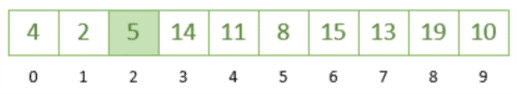Let’s take the leftmost partition (index 0 – 1) and repeat the steps. We’re going to choose number 2 as our pivot and rearrange accordingly which gives us the following: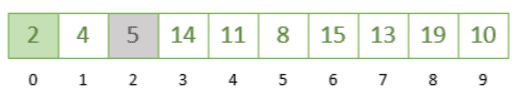Next, we take the rightmost partition (index 3 – 9) and take 10 as our pivot; any number greater than 10 will be moved to the right of it and the numbers smaller than 10 will go to its left: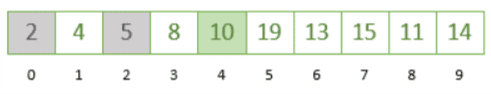As we can see by positioning each chosen pivot, we’re slowly getting closer to a sorted array! If we continue repeating the steps on the remaining partition from index 5 to 9, we’ll finally reach a point where our array is sorted from smallest to largest.

The image below shows all the steps together finally giving us a sorted array: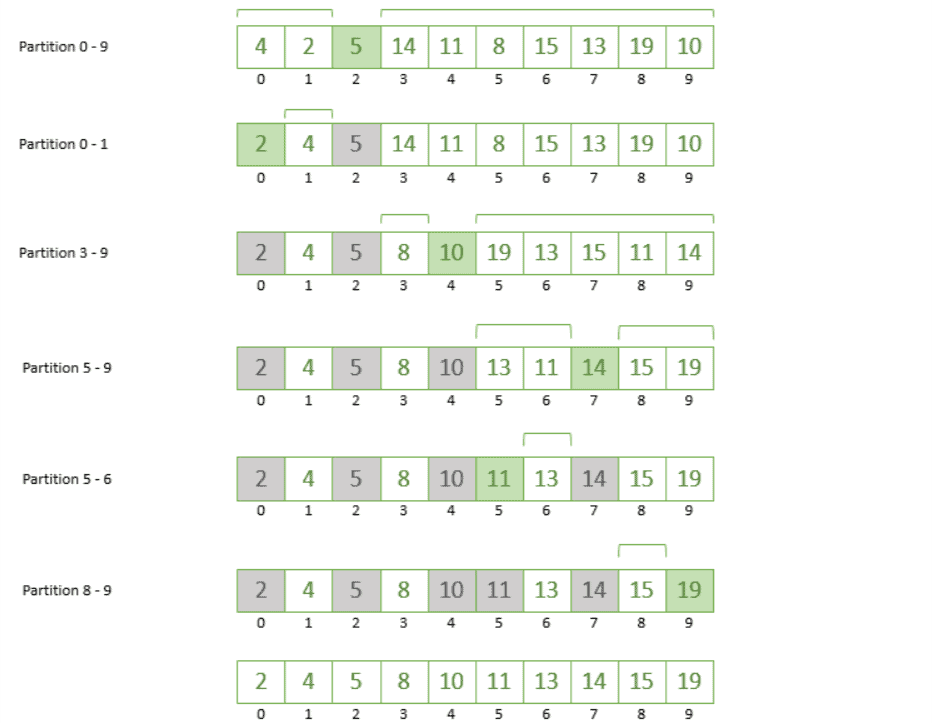## 3. Implementing Quicksort

In our previous example, we were doing the same logic recursively on smaller and smaller sub-arrays. But when it comes to writing this as an actual algorithm how do we actually implement it?

The most obvious way which comes to mind is that we create a new array on each step and copy things over each time. However, Quicksort is usually implemented as an in-place sorting algorithm. This means it operates on the actual array it’s sorting without creating too many additional variables which is great for memory usage.

### 3.1. Quicksort Pseudocode

Let’s see how implementing quicksort as an in-place sorting algorithm would look like in pseudo-code. The following is an implementation of the Quicksort algorithm using what’s known as the Hoare Partition scheme:If we look at the Partition function in this algorithm, we’ll find that this is where most of the logic resides.

First, we choose a pivot which in this case is the leftmost element of the array or sub-array we want to partition. Next, we use the variables i and j to traverse the array from both sides. The stopping condition for i is if we find something greater than the pivot and for j if we find an element less than the pivot.

Next, we check to see if i and I are on opposite sides of the array and have not overlapped. If that’s the case, then we can perform a swap operation that puts the element at index i in place of the element at index j and vice versa.

We’ll continue like this in a loop until we’ve swapped all elements around the pivot. Finally, we’ll reach a point were i and j overlap. At this point, we return from the Partition function with the value of j; this is the actual position were our pivot should be located and we use it, later on, to sort the left and right partitions by calling Quicksort(array, left, pivotPosition) and Quicksort(array, pivotPosition + 1, right).

### 3.2. Other Implementations of Quicksort

In our previous section, we saw how Quicksort could be implemented using a scheme known as the Hoare Partition scheme. However, there are actually other ways of implementing Quicksort such as the Lomunto Partition scheme.

There are many implementations of Quicksort which include elements of other sorting algorithms such as insertion sort and heap sort. There are also variations that use different ways of choosing the pivot such as dual-pivot Quicksort which is used in the Java Arrays.sort() methods for primitive data types. The famous Linq library in .Net also uses Quicksort by default with small variations.

## 4. Quicksort Analysis

In our first example, we were always picking the rightmost element in each sub-array as our pivot. However, we can actually pick a pivot in a number of ways:
• Picking a random element
• Always picking the rightmost
• Always picking the leftmost
• Picking the middle element

In most cases randomly picking a pivot is the preferred approach to increase the likelihood of a more even split of the partitions. An even split results in a best-case complexity of O(n log n).

On the other hand, an uneven split could have an adverse impact on the efficiency of Quicksort. For example, if we happened to pick the largest element as the pivot each time the subarrays would not be divided equally and all the elements would end up in the right sub-array. This would result in a worst-case complexity of O(n^2).

## 5. Conclusion

In this tutorial, we learned about the Quicksort algorithm for sorting elements. We found that Quicksort is quite popular because of its space and time efficiency.

Finally, we learned that Quicksort is most advantageous when implemented in-place with a randomly selected pivot.

Subscribe
Notify of